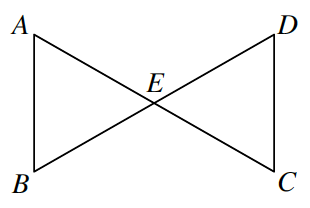### Home > GC > Chapter 7 > Lesson 7.2.2 > Problem7-61

7-61.

Jester started to prove that the triangles below are congruent. He is only told that point $E$ is the midpoint of segments $\overline{AC}$ and $\overline{BD}$.Copy and complete his flowchart below. Be sure that a reason is provided for every statement.

 $\enclose{circle}{\; \\ \qquad \text{E is a midpoint} \qquad \\}$ $\huge \swarrow$Bubble 1 has an arrow pointing down to bubble 3. $\huge \searrow$ Given Bubble 1 has an arrow pointing down to bubble 4. $\enclose{circle}{\; \\ \qquad \qquad \qquad \qquad \\}$Fill in bubble 2.Vertical angles are congruent. $\enclose{circle}{\; \\ \qquad \; \; \; \qquad \qquad \\}$Fill in bubble 3. $\enclose{circle}{\; \\ \qquad \overline{AE} \cong \overline{CE} \qquad \\}$A, E is congruent to C, E Definition of midpoint. $\huge \searrow$Bubble 2 has an arrow pointing down to bubble 5. $\huge \downarrow$Bubble 3 has an arrow pointing down to bubble 5. $\huge \swarrow$Bubble 4 has an arrow pointing down to bubble 5. $\enclose{circle}{\; \\ \qquad \ \ \ \qquad \qquad \\}$Fill in bubble 5

 $\enclose{circle}{\; \\ \qquad \text{E is a midpoint} \qquad \\}$ $\huge \swarrow$Bubble 1 has an arrow pointing down to bubble 3. $\huge \searrow$ Given Bubble 1 has an arrow pointing down to bubble 4. $\enclose{circle}{\; \\ \qquad \boldsymbol{\color{red}{\angle AEB \cong \angle CED}} \qquad \\}$Vertical angles are congruent. $\enclose{circle}{\; \\ \qquad \bf{\color{red}{\overline{BE}\cong \overline{DE}}} \qquad \\}$$\bf{\color{red}{\text{Definition of midpoint.}}}$ $\enclose{circle}{\; \\ \qquad \overline{AE} \cong \overline{CE} \qquad \\}$Definition of midpoint. $\huge \searrow$Bubble 2 has an arrow pointing down to bubble 5. $\huge \downarrow$Bubble 3 has an arrow pointing down to bubble 5. $\huge \swarrow$Bubble 4 has an arrow pointing down to bubble 5. $\enclose{circle}{\; \\ \qquad \bf{\color{red}{\triangle AEB \cong \triangle CED}} \qquad \\}$$\quad \quad \quad \quad \quad \quad \quad \bf{\color{red}{SAS \cong}}$